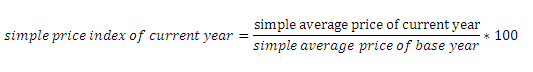# Simple price index number

Rice index number constructed giving equal weights to all commodities is called simple price index number, in this case, all commodities are given equal importance. The average price is calculated simply dividing the sum of prices by number of commodities.

Mathematically,

simple average price = sum of prices / no. of commodities

After the calculation of average price of base year and current year we change them into their relative values i.e. indices. The rice level of base year is treated as 100 and the price index of current year is calculated using the formula:Construction of simple price index number can be explained as following:

 commodities Price in 2011 Price in 2012 Rice Rs 35 Rs 40 Pulses Rs 30 Rs 95 Clothes Rs 250 Rs 275 Vegetables Rs 50 Rs 70 Firewood Rs 100 Rs 150 Total Rs515 Rs 630 Average (P/N) Rs 103 Rs 126 Price index 100 (126/103)*100 = 122.33

In the above table, we have taken only 5 commodities for the sake of simplicity. The sum of the prices of the commodities in the year 2012 is Rs 515 and in the year 2012 is Rs 630. If we divide this sum of prices by number of commodities we obtain the price level of the year RS 103 and Rs 126 respectively. If we change these price levels to price indices using the above formula we obtain 100 and 122.33. It shows there is rise in price level b 22.33 % in the year 2012.